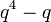# Number of conjugacy classes in general linear group of fixed degree over a finite field is polynomial function of field size

## Statement

Suppose$n$ is a natural number. Then, there exists a polynomial function$f$ of degree$n$ such that, for any prime power$q$, the number of conjugacy classes in the general linear group$GL(n,q)$ (i.e., the general linear group of degree$n$ over the finite field of size$q$) is$f(q)$.

## General observations

Below, we list some general observations about the polynomial in$q$ giving number of conjugacy classes in$GL(n,q)$.

Item Value
Degree of polynomial$n$
Leading coefficient of polynomial 1, i.e., it is always a monic polynomial
Factors of polynomial$q - 1$ is always a factor of the polynomial.
Coefficients of polynomial The polynomial is an integer-valued polynomial, i.e., it sends integers to integers. Is it also true that all coefficients of the polynomial are integers?

## Particular cases$n$ (degree of general linear group, degree of polynomial) polynomial of$q$ giving number of conjugacy classes in$GL(n,q)$ More information
1$q - 1$ This follows from the group being an abelian group of order$q - 1$. In fact, the group is a cyclic group, because multiplicative group of a finite field is cyclic, though we do not need that fact here.
2$q^2 - 1$ See element structure of general linear group of degree two over a finite field
3$q^3 - q$ See element structure of general linear group of degree three over a finite field
4$q^4 - q$ See element structure of general linear group of degree four over a finite field
5$q^5 - q^2 - q + 1$ See element structure of general linear group of degree five over a finite field
6$q^6 - q^2$ See element structure of general linear group of degree six over a finite field
7$q^7 - q^3 - q^2 + 1$

There is probably some general formula for these polynomials for all degrees without having to do the entire analysis of element structure and conjugacy classes, but it's unclear what this formula is.